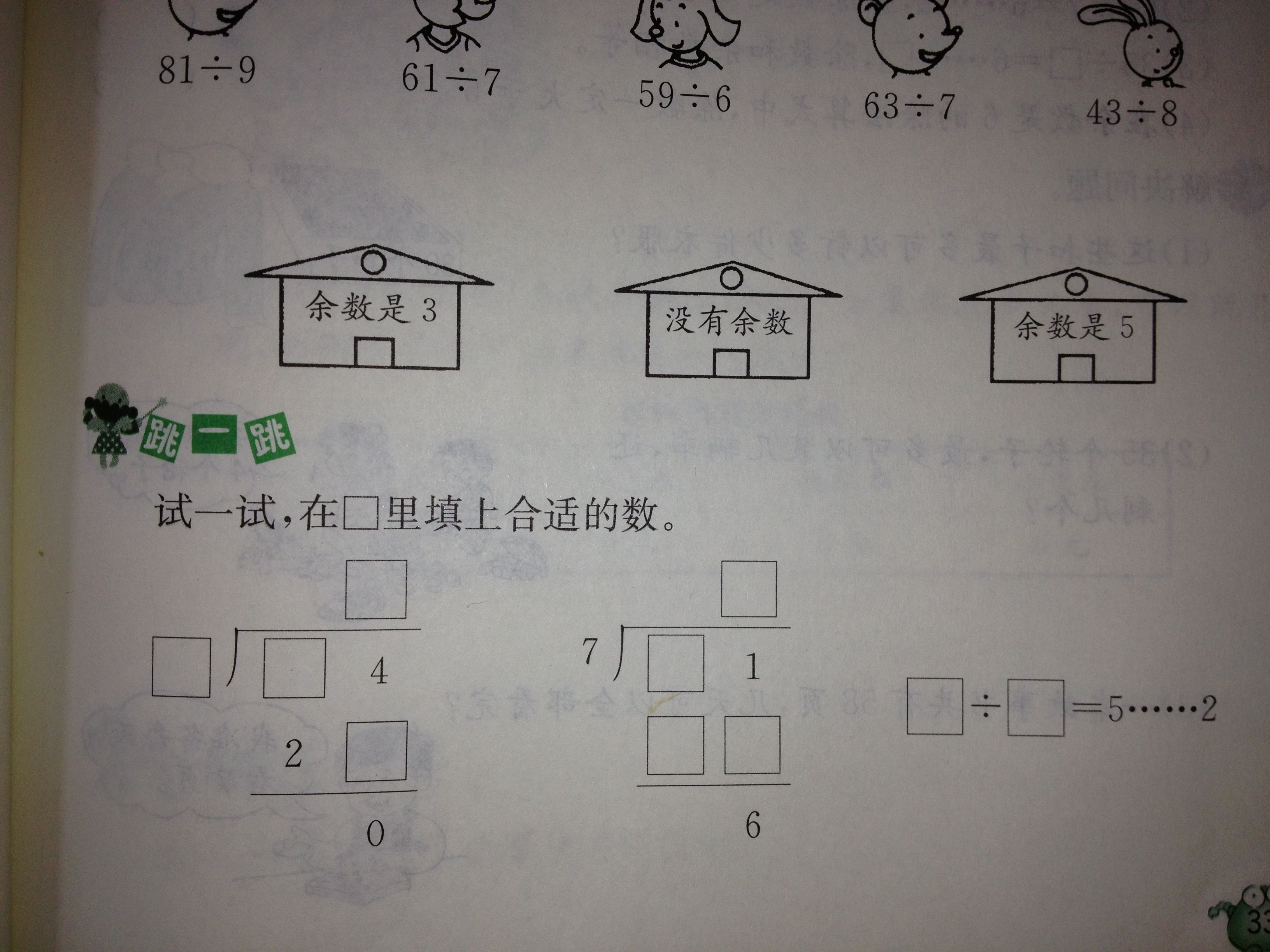## 二年级下册数学计算题总复习

3×6= 4×5= 6×2= 3×2= 6×6= 3＋5=

2×5= 4＋3= 4×6= 5×1= 6＋6= 2×2=

9－3×3= 5×5＋6= 5＋5×6= 27－4×2=

（ ）－6=42 （ ）＋9= 59 12－（ ）= 5　 45 + （ ）=100

50－（44＋6）= 43－6－30= 20＋（40－3）= 26－（13－7）=

71－37 34 77－59 48＋12 55－17 55－19

1. 48－19 28＋15 28－15 47－17 55－17

（ ）×5＜24 36＞（ ）×6 18＞（ ）×3

16+（ ）＜60 35 －（ ）＞27 5个5＞（ ）

17＋45＋26= 65－29＋36= 100－37－17=

75＋(53－28)= 100－（64+17）= 28＋35－16=

27＋25＋36= 75－(51－22)= 39+（61－29）

6×9= 36＋63= 68-45= 24÷3= 37+20= 8÷8=

4＋3×8= 9－4×2= 32÷4＋5= 45－8÷2= 47＋16-35=

60-（12-7）= 8×（3+4）= 90-（95-65）= 65－18－12= 8÷4×2=

63－40＋25 35＋4×8 27＋81÷9

6×8－24 36÷6×4 72÷（24－16）

8×7 21－12

5＋4=9

9×7=63

÷3

60－

35÷5= 28÷6= 50÷8= 63÷8=

57÷9= 25÷6= 23÷4= 71÷9=

46－8＝ 67－50＝ 75－20＝ 40＋30＝ 2×8÷4=

9＋50＝ 72＋9＝ 53＋40＝ 63＋30＝ 72÷9+6=

60＋40＝ 8×9－3＝ 100－40＝ 79－26＝ 4+5×3=

54÷9＝    7×8＝    29+30＝    36÷6＝   32÷4＝

4×9＝   35+45＝   100-50＝   49÷7+500 ＝  81÷9÷3=

9÷3○9+3 20+40○66 51-36○20 48÷6○48-8

3×8○56÷7 23+56○9×9 4×6○3×8 56○9×7

72○8=3○3 20○15=5○7 35○5=11○4 36○6=5○6

2○3=30○5 32○30=2 45○45=11 5○7=42○7

32+68 – 29= 9×9 -9 = 5×7+19 = 81÷9-9=

88-65 +27 49÷7 +31 31+（25-13）= 35÷7×4

48+16 90-25= 88-35 -270= 42+38-31

20-9= 36+24= 4×6= 54-0= 34-19= 3×6= 5×6=

4×6= 20-6= 3×3= 6÷2= 12÷2= 10÷5= 5÷5=

5÷1= 30÷6= 6×4= 24÷4= 6×7= 7÷1= 56÷7=

7÷7= 7×6+7= 7×4+4= 49÷7= 35÷5= 28÷4=

7×7= 14÷7= 7÷1= 7×8= 56÷7= 16-8= 42÷7=

63÷（ ）=9 8×（ ）=32 63－（ ）=30 5×（ ）=20

（ ）×2=6 17+（ ）=34 48－（ ）=19 7×5=（ ）

54－（ ）=21 29+（ ）=71 42+（ ）=75 48－（ ）=10

29=5×（ ）+4 60－（ ）=23 88－（ ）=24 47+（ ）=60

32-16+15 28+（18+15） 89-18-65 （27-19）×5

28÷4×6 56÷（16-9） 32÷4-5 5+4×6

27÷3= 7×5= 8×4= 7×8= 8×9= 6×9=

28÷7= 36÷4= 40÷8= 6×9= 7×7= 12÷4×5=

6×4÷3= 5×1×6= 3+3÷1=

30+30÷6 71－10+9 40－（8+5） 12＋12÷3

20－25÷5 32÷4+48÷6 16÷4÷2 5×6+60

54÷9 = 42-28 = 45÷6 = 50÷8 =

35+46= 36÷7 = 54÷9= 37-19=

（1）A + A + A = 6 （2）D + D +D +D =32

B + B + B = 12 D = （ ）

A + B =（ ）

42÷7＝ 9×5＝ 28÷4＝ 35÷7=

52＋32＝ 19＋17＝ 28＋9＝ 36＋5＝

28＋3＝ 16＋30= 28－6＝ 45÷9＋37＝

100－9×3＝ 80＋5×4＝ 30－36÷4＝

（1）5×6=30 （2） 43-36=7 （3）12+8=20

65-30=35 21 ÷7=3 20÷5=4

（1）8＋16÷8 改正： （2）10－5×2 改正 ：
＝24÷8 ＝5×2
＝3 ＝10

（1）63－（40＋20） （2）35＋4×8 （3）27＋81÷9

（4）6×8－24 （5）36÷6×4 （6）49÷7＋63

（ ）÷（ ）=5……2 （ ）÷（ ）=5……2 （ ）÷（ ）=5……2

63÷7= 72÷8＝ 40＋60＝ 93－26= 16÷4＝

58－57＝ 80－9＝ 35÷7＝ 70－50＝ 15÷3×5＝

53-24+38＝ 4×8＋6 ＝ 15－6×2＝ 18－8×2＝ 52－22＋9＝

47－12＋5 6×3+60 7×9-17 21÷7+14

＝ ＝ ＝ ＝

＝ ＝ ＝ ＝

6×8+15 （15+15）÷6 （75－68）×7 7×（45÷5）

＝ ＝ ＝ ＝

＝ ＝ ＝ ＝

（ ）×6<57 ( ) ×7<43 38>( ) ×5

( ) ×4<31 ( ) ×8<26 60>( ) ×9

63÷（ ）＜ 8 28 ＞ 6×（ ） 32÷4 ＞( )

35÷7 42÷6 7×7 72÷8 36÷6

（ ）> ( ) > ( ) >( ) >( )

（ ）×4＜37 8×（ ）＜70 54＞8×（ ） （ ）×6＜25

9×（ ）＜65 48＞5×( ) （ ）×6＜60 49＞（ ）×6

1. 13 ÷ 3，余数是( )。

2. 用一堆小棒摆 ，如果有剩余，可能会剩( )根小棒。

3. ○÷△= 8……7，△最小是( )。

4. B ÷ 9 = 7……□，余数最大是( )。

5. □÷ 7 = 6……□，余数最大是( )，这时被除数是( )。

21÷6＝ 19÷4＝ 67÷9＝ 38÷5＝ 52÷7＝

72÷8＝ 54—28= 25÷3＝ 8×9= 56－7=

17÷2＝ 43÷9＝ 84÷9＝ 27÷5＝ 9÷1=

64÷8= 36÷7= 100－28= 56÷9= 47÷6=

24÷4×2 4＋5×5 57－16＋4 68＋(37－19) 52－32÷45×3＝ 42÷7＝ 9×5＝ 28÷4＝ 6×3＝

35÷7 = 9×2＝ 8×3＝ 21＋32＝ 19＋17＝

28＋9＝ 36＋5＝ 28＋3＝ 16＋30＝ 28－6＝

13÷3＝ 45÷8＝ 9÷3＝ 21÷6＝ 36÷5＝

（1）9×8＋12 （2）20＋9×2 （3）72÷8＋30

（4）56－8×3 （5）21＋6×3 （6）15－40÷5

（1）63－40＋25= （2）35＋48 = （3）17＋81-9 =

（4）68－（24+9）= （5）36+（64 -18）= （6）49+7＋44=

（1）□÷7＝△……☆，□÷☆＝△……4，☆可能是（ ）。

（2）已知□÷□＝8……5 ，被除数最小是( )。

（3）（ ）÷5＝6……（ ） ，余数最大时，被除数是（ ）。

（4）8个碗，14根筷子，可以分给（　）个客人

14－6×2＝ 8＋5×3＝ 6×4－20＝ 30－5×4＝ （90－60）÷6＝

8×（7－4）＝ 36÷（3×3）＝ 16÷8×7＝ 80－5×8＝ 9×3＋20＝

26÷4= 27÷5= 42÷7= 82÷9= 35÷5=

5÷5= 5×5= 54÷9= 71÷8= 62÷9=

（1）（90－36）÷6 （2）37＋4×9 （3）73－（36＋18）

（4）56－8×3 （5）6×（49－40） （6）15－40÷5

（1） 8＋16÷8 改正： （2）40－15÷5 改正：
＝24÷8 ＝25÷5
＝3 ＝5
（3） 12÷2×3 改正： （4）（66—24）÷6 改正：

=12÷6 =66－4

=2 =62

（1） 64÷8=8 （2）68－40=28 （3）20－12=8

30＋8=38 28÷7=4 3×8=24

（ ）×8<57 ( ) ×6<43 38>( ) ×8

( ) ×7<31 ( ) ×4<26 50>( ) ×7

56÷（ ）＜ 8 50 ＞ 6×（ ） 81÷9 ＞( )

1、在□÷7中，如果有余数，余数可能是（ ）其中最大是（ ）。

2、在一个余数是8的除法算式中，除数最小是（ ）。

3、49里面最多有（ ）个9，余数是（ ）。

4、19根小棒可以摆（ ）个正方形，还剩（ ）根。